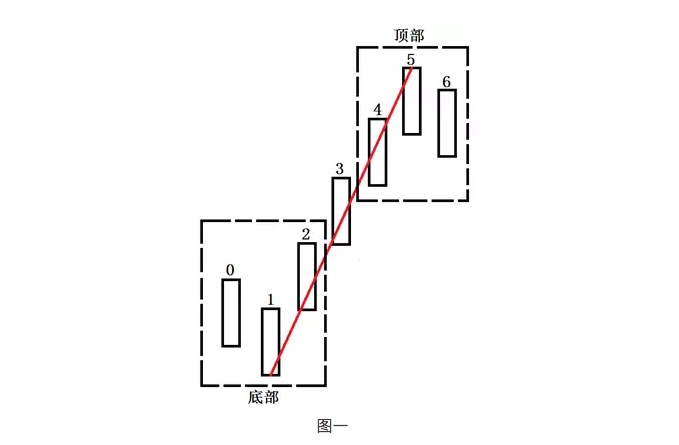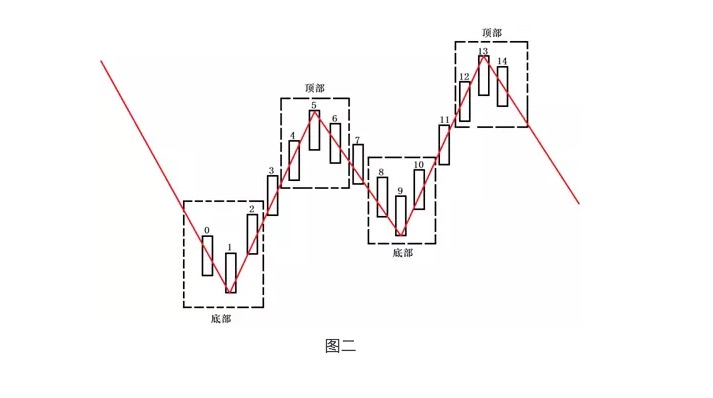# 对均线系统的重新思考

Author: 小小梦, Created: 2016-11-30 11:51:51, Updated: 2016-11-30 11:56:27

• ### 2、何为趋势• ### 3、均线的重新设置

``````一段周线行情
= 至少有5根周K线构成
= 至少有25根日K线构成（一周5个交易日）
= 至少有100根60分钟K线构成（一天4个小时）

= 日线一定形成新的趋势
= 至少有13根日K线构成
= 至少有52根60分钟K线构成

= 至少有5根日K线构成
= 至少有20根60分钟K线构成

= 60分钟一定形成新的趋势
= 至少有13根60分钟K线构成

=至少有5根60分钟K线构成

MA1 = 5； MA2 = 13； MA3 = 20； MA4 = 52；MA5 = 100；

MA1 = 5；MA2 = 13；MA3 = 20；MA4 = 52；MA5 = 60；

MA1 = 5；MA2 = 13；MA3 = 25；MA4 = 65；MA5 = 100；

M1 = 5；MA2 = 13；MA3 = 20；MA4 = 52；MA5 = 80；

MA1 = 5；MA2 = 13；MA3 = 40；MA4 = 104；MA5 = 200；

MA1 = 5；MA2 = 13；MA3 = 30；MA4 = 78；MA5 = 240；
``````
• ### 4、结论

MA1描述本级别的一段行情，MA2描述本级别的趋势，MA3描述高一级别的一段行情，MA4描述高一级别的趋势，MA5描述高两个级别的一段行情，这样就能在不同级别的图形上，将更高级别的均线刻画在小级别图形上，使大小级别均线有机地当做一个整体来看待，均线系统的有效性也将提升。

More